# Convex polyhedron

The convex hull of a finite number of points in a Euclidean space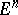. Such a convex polyhedron is the bounded intersection of a finite number of closed half-spaces. An infinite convex polyhedron is the intersection of a finite number of closed half-spaces containing at least one ray; the spaceis also conventionally considered to be a convex polyhedron. In this sense a convex polyhedron is the closed convex hull of a finite number of points and rays. The dimension of a convex polyhedron is the minimal dimension of the spacethat contains it.

A convex polyhedron is a special case of a convex set. Being an intersection of half-spaces, a convex polyhedron is described by a system of linear inequalities and may be studied by algebraic tools. The methods of minimization of linear forms on a convex polyhedron form the subject of linear programming.

A convex polyhedron has a finite number of faces (intersections of the convex polyhedron with the supporting hyperplanes). Each face of a convex polyhedron is a convex polyhedron of lower dimension. Faces of the faces are also faces of the original polyhedron. One-dimensional faces are known as edges; zero-dimensional faces are known as vertices. A bounded convex polyhedron is the convex hull of its vertices.

In the theory of convex surfaces (cf. Convex surface) the boundary of a convex polyhedron, and sometimes a part of such a boundary, is called a convex polyhedron . In the latter case one speaks of a convex polyhedron with boundary. In elementary geometry it is accepted to define a polyhedron as a figure composed of polygons in a special manner , after which a convex polyhedron is defined as a polyhedron lying on one side of the plane passing through any one of its-dimensional faces.

A bounded-dimensional convex polyhedron has not fewer thanvertices. The simplest structure is that of a simplex, which hasvertices. Any bounded convex polyhedron can be subdivided into simplices with adjacent common faces.

In the Euclidean space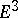there are five regular convex polyhedra: the tetrahedron, the cube, the octahedron, the dodecahedron, and the icosahedron. For their properties and their analogues see Regular polyhedra; Semi-regular polyhedra. For convex polyhedra with special structural features see Isogons and isohedra; Zonohedron. Special types of convex polyhedra — stereohedra, parallelohedra, planigons (cf. Stereohedron; Parallelohedron; Planigon) — are connected with regular subdivisions of space.

The possible types of structure of the network of faces of convex polyhedra have not been exhaustively studied. Letbe the number of-dimensional faces of a bounded-dimensional convex polyhedron; then Euler's relation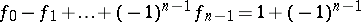applies. It has a topological nature: It is valid for any subdivision of the sphere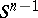into simple cells. If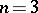, it is possible to find a convex polyhedron with such a network structure in the Euclidean spacefor any connected network of faces on the sphere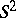which does not form dihedral and self-intersecting cells (Steinitz's theorem). If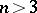, the structure of the network of faces of a convex polyhedron is less arbitrary than the possible subdivisions of the sphere . Specific extremal problems, involving the structure of the network of faces, the number of edges or their overall length, etc., may be posed in the class of convex polyhedra .

Approximation of convex bodies by convex polyhedra is a universal method of investigation. Many results in mixed-volume theory, existence theorems, uniqueness theorems, stability theorems for convex surfaces with fixed parameters, and a geometric method of solving the Monge–Ampère equation have been obtained in this way. The effectiveness of this method is due to the fact that a convex polyhedron is defined by a finite number of data; the general theorems can be simply formulated for convex polyhedra; synthetic methods of investigation are applicable to convex polyhedra.

A large part of the theory of convex polyhedra has been formulated in the context of the theory of surfaces . In the Euclidean spacetwo bounded convex polyhedra with identical faces in the same order can be brought into congruence by a shift (Cauchy's theorem). In, for certain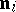,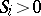which satisfy the relationship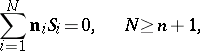there exists a unique, up to a shift, convex polyhedron with exterior unit normalsto the faces, and with face areas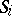(Minkowski's theorem). An evolvent of planar polygons, glued together so that the result is homeomorphic to the sphereand such that the sum of the angles pieced together around each vertex is smaller than or equal to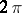, is isometric to a convex polyhedron in, and this convex polyhedron is unique up to a shift (Aleksandrov's theorem). Two convex polyhedra incan be brought into congruence by a shift if for every normal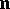the faces (with exterior normal) cannot be brought by shifting into a position such that one of this faces is part of the other, but does not coincide with it. For rays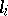issuing from a point and for numbers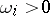there exists a unique, up to a homothety, convex polyhedron with vertices on the raysand curvatures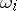at these vertices.